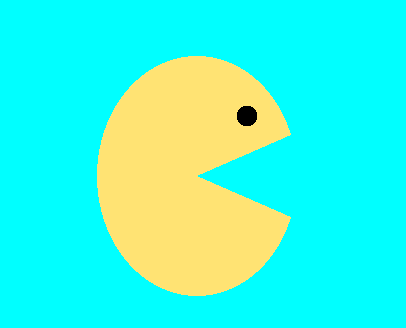# PacMan drawing! got stuck

Hey, I am a new OpenGL user. trying to learn it.
here I tried to draw PacMan.but got stuck while trying to shut and open PacMan’s mouth. please help me out.
I am using Triangle_Fan for draw the circle using a loop.Could you share the code for rendering the pacman ?

Try the following code :

``````glColor4f(1f, 1f, 0f, 1f);

int centerx = 1000;
int centery = 500;

float angleMouth = 30f * 3.1415926f / 180.0f; //(in radians)

glBegin(GL_TRIANGLE_FAN);
glVertex(centerx, centery);
for(int i = 0; i < 100; i++) {
float angle = angleMouth/2f + (3.1415926f * 2.0f - angleMouth) * (float) i / (float) 99.0f;
glVertex(centerx + 100 * Math.cos(angle), centery + 100 * Math.sin(angle));
}
glEnd();
``````

Change the `angleMouth` parameter to change the mouth opening. (note that this is written in JOGL, use the appropriate sine and cosine functions for your application).

Finally, these are the vertex functions :

``````private void glVertex(double x, double y) {
gl.glVertex2d(getXScreen(x), getYScreen(y));
}

double getXScreen(double x) {
return 2f * (x + 0.5) / (double) panel.getWidth() - 1f;
}

double getYScreen(double y) {
return 2f * (y + 0.5) / (double) panel.getHeight() - 1f;
}
``````

Again, this is in java, use the appropriate equivalent synthax in whatever language you’re using